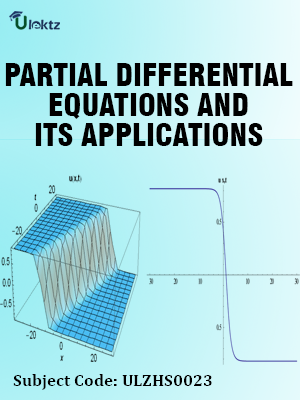•My WalletMy Order
•My Profile
•My Connections
•My Books
•My Videos
•My Tests
•My Calender
•My Messages
•My Shopping Cart
•My Orders
•Account Settings
•Help

# Book Details# Partial Differential Equations and Its Applications

 Course Code : ULZHS0023 Author : uLektz University : General for All University Regulation : 2013 Categories : Engineering Mathematics Format :ePUB3 (DRM Protected) Type : eBook

FREE

Description :Partial Differential Equations and Its Applications of ULZHS0023 covers the latest syllabus prescribed by General for All University for regulation 2013. Author: uLektz, Published by uLektz Learning Solutions Private Limited.

Note : No printed book. Only ebook. Access eBook using uLektz apps for Android, iOS and Windows Desktop PC.

##### Topics
###### UNIT I PARTIAL DIFFERENTIAL EQUATIONS AND ITS APPLICATIONS

1. Introduction- Homogeneous function-Euler’s theorem

2. Total derivative-Chain rule

3. Generalized Mean value theorem for single variable (without proof)

4. Taylor’s and Mc Laurent’s series expansion of functions of two variables- Functional dependence

5. Jacobian

6. Applications: Maxima and Minima of functions of two variables without constraints and Lagrange’s method (with constraints)

7. Limits and Continuity

8. Lagrange‟s method of undetermined multipliers.

9. FIRST ORDER PARTIAL DIFFERENTIAL EQUATIONS: Formation of partial differential equations by elimination of arbitrary constants and arbitrary functions

10. Solutions of first order linear (Lagrange) equation and nonlinear (standard types) equations

11. HIGHER ORDER PARTIAL DIFFERENTIAL EQUATIONS: Solutions of Linear Partial differential equations with constant coefficients.

12. RHS term of the type eax + by,sin(ax + by), cos(ax + by), xm yn - Classification of second order partial differential equations

13. APPLICATIONS: Method of separation of Variables

14. Solution of One dimensional Wave equation

15. Solution of One dimensional Heat equation, One dimensional heat equation with the initial and boundary conditions

16. Two-dimensional Laplace equation

### Related Books